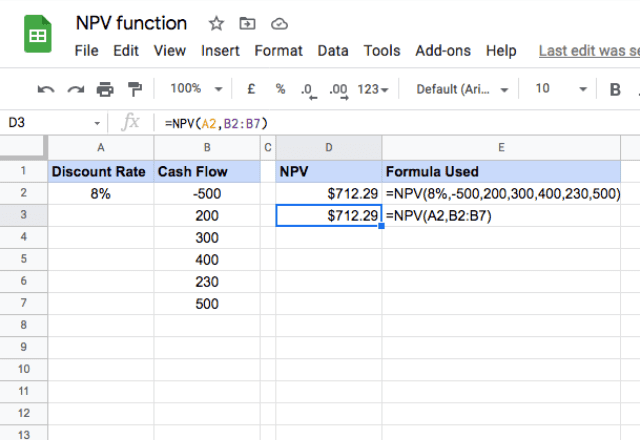# How to use the NPV formula in Google SheetsGoogle Sheets is a great tool to perform financial calculations and analysis with. A typical example of this is that you might want to find the today’s value of an investment that will pay off in the future. The NPV formula in Google Sheets helps with just that. All you need to have is a series of periodic cash flows and the discount rate that will be used to bring them back to today’s value.

### Syntax

=NPV(discount, cashflow1, [cashflow2, …])
• discount – is the discount rate of the investment over a single period.
• cashflow1 – is the value of the first future cash flow – either positive or negative.
• cashflow2, … – [OPTIONAL] – the subsequent values of cash flows in the future.

### What is NPV?

NPV stands for Net Present Value. The concept of NPV is all about the time value of money – in short, money today is worth more than money in the future. That’s not a complicated concept: if someone offered you a choice between \$100 today and \$100 a year from now, you’d probably choose to get the money today. The degree to which the value of money changes over time is the discount rate. The higher the discount rate, the less valuable money is in the future.

NPV takes a series of cash flows and discounts them back to the present value (i.e. what they’re worth today). The further in the future the cash flow is, the lower its present value. When you discount each cash flow and add them all up, you get the overall NPV.

### Usage: NPV formula in Google Sheets

Now that you’ve seen the syntax of the NPV function, and understand what NPV actually means, I’ll show an example to reinforce how the function is used.

Please take a look at the snapshot below.In this example, you have a \$500 investment up front (denoted by the negative sign), and then a series of payments. At a discount rate of 8%, the NPV of this series of cash flows is \$712.29.

You can either type the values into the function (as done in the first example) or just input the cell references and ranges that contain the values. The last method is generally easier.

### Things to keep in mind

• The NPV function considers the cash flows in the order they are referenced, so make sure the order is correct.
• The time periods must be consistent. You can use any time period you want (months, weeks, years, minutes… anything!) as long as it’s consistent – that means each cash flow is the same amount of time from the next.
• If you have a series of cash flows that don’t follow a consistent time period, you should use the XNPV function.
• The discount rate must be based on the same time period as the cash flows. This means that, if your cash flows are on a monthly basis, the discount rate should also be a monthly rate.
• A negative cash flow represents a payment from the investor (i.e. money going out). Whereas a positive value represents the investor receiving income (i.e. money coming in).
• Use the PV formula for cash flows that have constant amounts. On the contrary, the NPV formula can handle varying cash flow values.

Editor’s note: This is a revised version of a previous post that has been updated for accuracy and comprehensiveness.